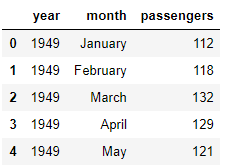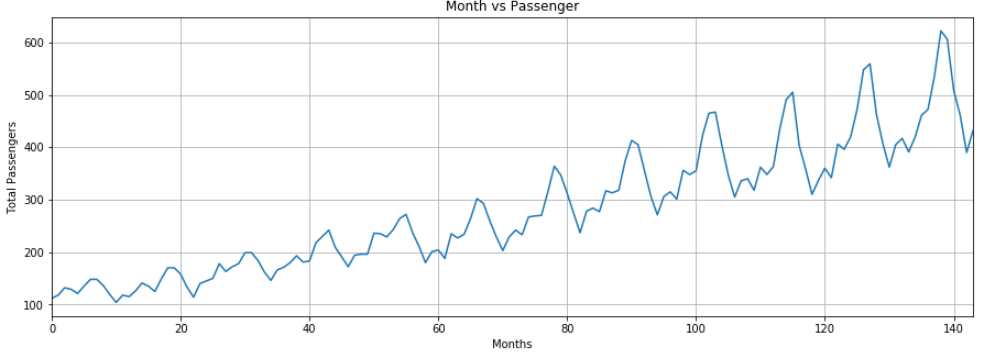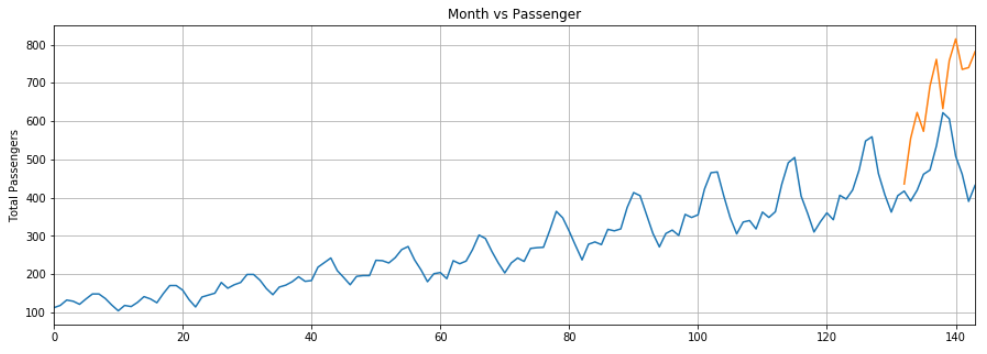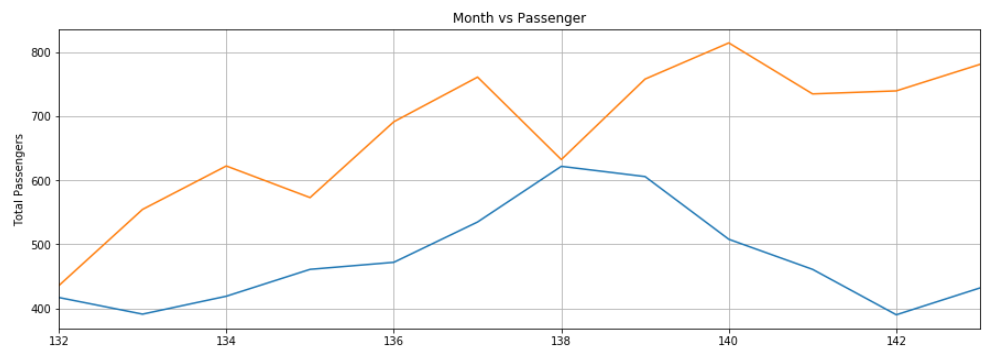Time Series Prediction using LSTM with PyTorch in Python - Stack Abuse

# Time Series Prediction using LSTM with PyTorch in PythonTime series data, as the name suggests is a type of data that changes with time. For instance, the temperature in a 24-hour time period, the price of various products in a month, the stock prices of a particular company in a year. Advanced deep learning models such as Long Short Term Memory Networks (LSTM), are capable of capturing patterns in the time series data, and therefore can be used to make predictions regarding the future trend of the data. In this article, you will see how to use LSTM algorithm to make future predictions using time series data.

In one of my earlier articles, I explained how to perform time series analysis using LSTM in the Keras library in order to predict future stock prices. In this article, we will be using the PyTorch library, which is one of the most commonly used Python libraries for deep learning.

Before you proceed, it is assumed that you have intermediate level proficiency with the Python programming language and you have installed the PyTorch library. Also, know-how of basic machine learning concepts and deep learning concepts will help. If you have not installed PyTorch, you can do so with the following pip command:

\$ pip install pytorch


### Dataset and Problem Definition

The dataset that we will be using comes built-in with the Python Seaborn Library. Let's import the required libraries first and then will import the dataset:

import torch
import torch.nn as nn

import seaborn as sns
import numpy as np
import pandas as pd
import matplotlib.pyplot as plt
%matplotlib inline


Let's print the list of all the datasets that come built-in with the Seaborn library:

sns.get_dataset_names()


Output:

['anscombe',
'attention',
'brain_networks',
'car_crashes',
'diamonds',
'dots',
'exercise',
'flights',
'fmri',
'gammas',
'iris',
'mpg',
'planets',
'tips',
'titanic']


The dataset that we will be using is the flights dataset. Let's load the dataset into our application and see how it looks:

flight_data = sns.load_dataset("flights")
flight_data.head()


Output:The dataset has three columns: year, month, and passengers. The passengers column contains the total number of traveling passengers in a specified month. Let's plot the shape of our dataset:

flight_data.shape


Output:

(144, 3)


You can see that there are 144 rows and 3 columns in the dataset, which means that the dataset contains 12 year traveling record of the passengers.

The task is to predict the number of passengers who traveled in the last 12 months based on first 132 months. Remember that we have a record of 144 months, which means that the data from the first 132 months will be used to train our LSTM model, whereas the model performance will be evaluated using the values from the last 12 months.

Let's plot the frequency of the passengers traveling per month. The following script increases the default plot size:

fig_size = plt.rcParams["figure.figsize"]
fig_size = 15
fig_size = 5
plt.rcParams["figure.figsize"] = fig_size


And this next script plots the monthly frequency of the number of passengers:

plt.title('Month vs Passenger')
plt.ylabel('Total Passengers')
plt.xlabel('Months')
plt.grid(True)
plt.autoscale(axis='x',tight=True)
plt.plot(flight_data['passengers'])


Output:The output shows that over the years the average number of passengers traveling by air increased. The number of passengers traveling within a year fluctuates, which makes sense because during summer or winter vacations, the number of traveling passengers increases compared to the other parts of the year.

### Data Preprocessing

The types of the columns in our dataset is object, as shown by the following code:

flight_data.columns


Output:

Index(['year', 'month', 'passengers'], dtype='object')


The first preprocessing step is to change the type of the passengers column to float.

all_data = flight_data['passengers'].values.astype(float)


Now if you print the all_data numpy array, you should see the following floating type values:

print(all_data)


Output:

[112. 118. 132. 129. 121. 135. 148. 148. 136. 119. 104. 118. 115. 126.
141. 135. 125. 149. 170. 170. 158. 133. 114. 140. 145. 150. 178. 163.
172. 178. 199. 199. 184. 162. 146. 166. 171. 180. 193. 181. 183. 218.
230. 242. 209. 191. 172. 194. 196. 196. 236. 235. 229. 243. 264. 272.
237. 211. 180. 201. 204. 188. 235. 227. 234. 264. 302. 293. 259. 229.
203. 229. 242. 233. 267. 269. 270. 315. 364. 347. 312. 274. 237. 278.
284. 277. 317. 313. 318. 374. 413. 405. 355. 306. 271. 306. 315. 301.
356. 348. 355. 422. 465. 467. 404. 347. 305. 336. 340. 318. 362. 348.
363. 435. 491. 505. 404. 359. 310. 337. 360. 342. 406. 396. 420. 472.
548. 559. 463. 407. 362. 405. 417. 391. 419. 461. 472. 535. 622. 606.
508. 461. 390. 432.]


Next, we will divide our data set into training and test sets. The LSTM algorithm will be trained on the training set. The model will then be used to make predictions on the test set. The predictions will be compared with the actual values in the test set to evaluate the performance of the trained model.

The first 132 records will be used to train the model and the last 12 records will be used as a test set. The following script divides the data into training and test sets.

test_data_size = 12

train_data = all_data[:-test_data_size]
test_data = all_data[-test_data_size:]


Let's now print the length of the test and train sets:

print(len(train_data))
print(len(test_data))


Output:

132
12


If you now print the test data, you will see it contains last 12 records from the all_data numpy array:

print(test_data)


Output:

[417. 391. 419. 461. 472. 535. 622. 606. 508. 461. 390. 432.]


Our dataset is not normalized at the moment. The total number of passengers in the initial years is far less compared to the total number of passengers in the later years. It is very important to normalize the data for time series predictions. We will perform min/max scaling on the dataset which normalizes the data within a certain range of minimum and maximum values. We will be using the MinMaxScaler class from the sklearn.preprocessing module to scale our data. For further details of the min/max scaler implementation, visit this link.

The following code normalizes our data using the min/max scaler with minimum and maximum values of -1 and 1, respectively.

from sklearn.preprocessing import MinMaxScaler

scaler = MinMaxScaler(feature_range=(-1, 1))
train_data_normalized = scaler.fit_transform(train_data .reshape(-1, 1))


Let's now print the first 5 and last 5 records of our normalized train data.

print(train_data_normalized[:5])
print(train_data_normalized[-5:])


Output:

[[-0.96483516]
[-0.93846154]
[-0.87692308]
[-0.89010989]
[-0.92527473]]
[[1.        ]
[0.57802198]
[0.33186813]
[0.13406593]
[0.32307692]]


You can see that the dataset values are now between -1 and 1.

It is important to mention here that data normalization is only applied on the training data and not on the test data. If normalization is applied on the test data, there is a chance that some information will be leaked from training set into the test set.

The next step is to convert our dataset into tensors since PyTorch models are trained using tensors. To convert the dataset into tensors, we can simply pass our dataset to the constructor of the FloatTensor object, as shown below:

train_data_normalized = torch.FloatTensor(train_data_normalized).view(-1)


The final preprocessing step is to convert our training data into sequences and corresponding labels.

## Better understand your data with visualizations.

•  30-day no-questions money-back guarantee
•  Beginner to Advanced
•  Updated regularly (latest update June 2021)
•  Updated with bonus resources and guides

You can use any sequence length and it depends upon the domain knowledge. However, in our dataset it is convenient to use a sequence length of 12 since we have monthly data and there are 12 months in a year. If we had daily data, a better sequence length would have been 365, i.e. the number of days in a year. Therefore, we will set the input sequence length for training to 12.

train_window = 12


Next, we will define a function named create_inout_sequences. The function will accept the raw input data and will return a list of tuples. In each tuple, the first element will contain list of 12 items corresponding to the number of passengers traveling in 12 months, the second tuple element will contain one item i.e. the number of passengers in the 12+1st month.

def create_inout_sequences(input_data, tw):
inout_seq = []
L = len(input_data)
for i in range(L-tw):
train_seq = input_data[i:i+tw]
train_label = input_data[i+tw:i+tw+1]
inout_seq.append((train_seq ,train_label))
return inout_seq


Execute the following script to create sequences and corresponding labels for training:

train_inout_seq = create_inout_sequences(train_data_normalized, train_window)


If you print the length of the train_inout_seq list, you will see that it contains 120 items. This is because though the training set contains 132 elements, the sequence length is 12, which means that the first sequence consists of the first 12 items and the 13th item is the label for the first sequence. Similarly, the second sequence starts from the second item and ends at the 13th item, whereas the 14th item is the label for the second sequence and so on.

Let's now print the first 5 items of the train_inout_seq list:

train_inout_seq[:5]


Output:

[(tensor([-0.9648, -0.9385, -0.8769, -0.8901, -0.9253, -0.8637, -0.8066, -0.8066,
-0.8593, -0.9341, -1.0000, -0.9385]), tensor([-0.9516])),
(tensor([-0.9385, -0.8769, -0.8901, -0.9253, -0.8637, -0.8066, -0.8066, -0.8593,
-0.9341, -1.0000, -0.9385, -0.9516]),
tensor([-0.9033])),
(tensor([-0.8769, -0.8901, -0.9253, -0.8637, -0.8066, -0.8066, -0.8593, -0.9341,
-1.0000, -0.9385, -0.9516, -0.9033]), tensor([-0.8374])),
(tensor([-0.8901, -0.9253, -0.8637, -0.8066, -0.8066, -0.8593, -0.9341, -1.0000,
-0.9385, -0.9516, -0.9033, -0.8374]), tensor([-0.8637])),
(tensor([-0.9253, -0.8637, -0.8066, -0.8066, -0.8593, -0.9341, -1.0000, -0.9385,
-0.9516, -0.9033, -0.8374, -0.8637]), tensor([-0.9077]))]


You can see that each item is a tuple where the first element consists of the 12 items of a sequence, and the second tuple element contains the corresponding label.

### Creating LSTM Model

We have preprocessed the data, now is the time to train our model. We will define a class LSTM, which inherits from nn.Module class of the PyTorch library. Check out my last article to see how to create a classification model with PyTorch. That article will help you understand what is happening in the following code.

class LSTM(nn.Module):
def __init__(self, input_size=1, hidden_layer_size=100, output_size=1):
super().__init__()
self.hidden_layer_size = hidden_layer_size

self.lstm = nn.LSTM(input_size, hidden_layer_size)

self.linear = nn.Linear(hidden_layer_size, output_size)

self.hidden_cell = (torch.zeros(1,1,self.hidden_layer_size),
torch.zeros(1,1,self.hidden_layer_size))

def forward(self, input_seq):
lstm_out, self.hidden_cell = self.lstm(input_seq.view(len(input_seq) ,1, -1), self.hidden_cell)
predictions = self.linear(lstm_out.view(len(input_seq), -1))
return predictions[-1]


Let me summarize what is happening in the above code. The constructor of the LSTM class accepts three parameters:

1. input_size: Corresponds to the number of features in the input. Though our sequence length is 12, for each month we have only 1 value i.e. total number of passengers, therefore the input size will be 1.
2. hidden_layer_size: Specifies the number of hidden layers along with the number of neurons in each layer. We will have one layer of 100 neurons.
3. output_size: The number of items in the output, since we want to predict the number of passengers for 1 month in the future, the output size will be 1.

Next, in the constructor we create variables hidden_layer_size, lstm, linear, and hidden_cell. LSTM algorithm accepts three inputs: previous hidden state, previous cell state and current input. The hidden_cell variable contains the previous hidden and cell state. The lstm and linear layer variables are used to create the LSTM and linear layers.

Inside the forward method, the input_seq is passed as a parameter, which is first passed through the lstm layer. The output of the lstm layer is the hidden and cell states at current time step, along with the output. The output from the lstm layer is passed to the linear layer. The predicted number of passengers is stored in the last item of the predictions list, which is returned to the calling function.

The next step is to create an object of the LSTM() class, define a loss function and the optimizer. Since, we are solving a classification problem, we will use the cross entropy loss. For the optimizer function, we will use the adam optimizer.

model = LSTM()
loss_function = nn.MSELoss()
optimizer = torch.optim.Adam(model.parameters(), lr=0.001)


Let's print our model:

print(model)


Output:

LSTM(
(lstm): LSTM(1, 100)
(linear): Linear(in_features=100, out_features=1, bias=True)
)


### Training the Model

We will train our model for 150 epochs. You can try with more epochs if you want. The loss will be printed after every 25 epochs.

epochs = 150

for i in range(epochs):
for seq, labels in train_inout_seq:
optimizer.zero_grad()
model.hidden_cell = (torch.zeros(1, 1, model.hidden_layer_size),
torch.zeros(1, 1, model.hidden_layer_size))

y_pred = model(seq)

single_loss = loss_function(y_pred, labels)
single_loss.backward()
optimizer.step()

if i%25 == 1:
print(f'epoch: {i:3} loss: {single_loss.item():10.8f}')

print(f'epoch: {i:3} loss: {single_loss.item():10.10f}')


Output:

epoch:   1 loss: 0.00517058
epoch:  26 loss: 0.00390285
epoch:  51 loss: 0.00473305
epoch:  76 loss: 0.00187001
epoch: 101 loss: 0.00000075
epoch: 126 loss: 0.00608046
epoch: 149 loss: 0.0004329932


You may get different values since by default weights are initialized randomly in a PyTorch neural network.

### Making Predictions

Now that our model is trained, we can start to make predictions. Since our test set contains the passenger data for the last 12 months and our model is trained to make predictions using a sequence length of 12. We will first filter the last 12 values from the training set:

fut_pred = 12

test_inputs = train_data_normalized[-train_window:].tolist()
print(test_inputs)


Output:

[0.12527473270893097, 0.04615384712815285, 0.3274725377559662, 0.2835164964199066, 0.3890109956264496, 0.6175824403762817, 0.9516483545303345, 1.0, 0.5780220031738281, 0.33186814188957214, 0.13406594097614288, 0.32307693362236023]


You can compare the above values with the last 12 values of the train_data_normalized data list.

Initially the test_inputs item will contain 12 items. Inside a for loop these 12 items will be used to make predictions about the first item from the test set i.e. the item number 133. The predict value will then be appended to the test_inputs list. During the second iteration, again the last 12 items will be used as input and a new prediction will be made which will then be appended to the test_inputs list again. The for loop will execute for 12 times since there are 12 elements in the test set. At the end of the loop the test_inputs list will contain 24 items. The last 12 items will be the predicted values for the test set.

The following script is used to make predictions:

model.eval()

for i in range(fut_pred):
seq = torch.FloatTensor(test_inputs[-train_window:])
with torch.no_grad():
model.hidden = (torch.zeros(1, 1, model.hidden_layer_size),
torch.zeros(1, 1, model.hidden_layer_size))
test_inputs.append(model(seq).item())


If you print the length of the test_inputs list, you will see it contains 24 items. The last 12 predicted items can be printed as follows:

test_inputs[fut_pred:]


Output:

[0.4574652910232544,
0.9810629487037659,
1.279405951499939,
1.0621851682662964,
1.5830546617507935,
1.8899496793746948,
1.323508620262146,
1.8764172792434692,
2.1249167919158936,
1.7745600938796997,
1.7952896356582642,
1.977765679359436]


It is pertinent to mention again that you may get different values depending upon the weights used for training the LSTM.

Since we normalized the dataset for training, the predicted values are also normalized. We need to convert the normalized predicted values into actual predicted values. We can do so by passing the normalized values to the inverse_transform method of the min/max scaler object that we used to normalize our dataset.

actual_predictions = scaler.inverse_transform(np.array(test_inputs[train_window:] ).reshape(-1, 1))
print(actual_predictions)


Output:

[[435.57335371]
[554.69182083]
[622.56485397]
[573.14712578]
[691.64493555]
[761.46355206]
[632.59821111]
[758.38493103]
[814.91857016]
[735.21242136]
[739.92839211]
[781.44169205]]


Let's now plot the predicted values against the actual values. Look at the following code:

x = np.arange(132, 144, 1)
print(x)


Output:

[132 133 134 135 136 137 138 139 140 141 142 143]


In the script above we create a list that contains numeric values for the last 12 months. The first month has an index value of 0, therefore the last month will be at index 143.

In the following script, we will plot the total number of passengers for 144 months, along with the predicted number of passengers for the last 12 months.

plt.title('Month vs Passenger')
plt.ylabel('Total Passengers')
plt.grid(True)
plt.autoscale(axis='x', tight=True)
plt.plot(flight_data['passengers'])
plt.plot(x,actual_predictions)
plt.show()


Output:The predictions made by our LSTM are depicted by the orange line. You can see that our algorithm is not too accurate but still it has been able to capture upward trend for total number of passengers traveling in the last 12 months along with occasional fluctuations. You can try with a greater number of epochs and with a higher number of neurons in the LSTM layer to see if you can get better performance.

To have a better view of the output, we can plot the actual and predicted number of passengers for the last 12 months as follows:

plt.title('Month vs Passenger')
plt.ylabel('Total Passengers')
plt.grid(True)
plt.autoscale(axis='x', tight=True)

plt.plot(flight_data['passengers'][-train_window:])
plt.plot(x,actual_predictions)
plt.show()


Output:Again, the predictions are not very accurate but the algorithm was able to capture the trend that the number of passengers in the future months should be higher than the previous months with occasional fluctuations.

### Conclusion

LSTM is one of the most widely used algorithm to solve sequence problems. In this article we saw how to make future predictions using time series data with LSTM. You also saw how to implement LSTM with PyTorch library and then how to plot predicted results against actual values to see how well the trained algorithm is performing.

Last Updated: February 2nd, 2020

## Improve your dev skills!

Get tutorials, guides, and dev jobs in your inbox.

No spam ever. Unsubscribe at any time. Read our Privacy Policy.

Usman MalikAuthor

Programmer | Blogger | Data Science Enthusiast | PhD To Be | Arsenal FC for Life

# Prepping for an interview?

• Improve your skills by solving one coding problem every day
• Get the solutions the next morning via email
• Practice on actual problems asked by top companies, like:

© 2013-2021 Stack Abuse. All rights reserved.